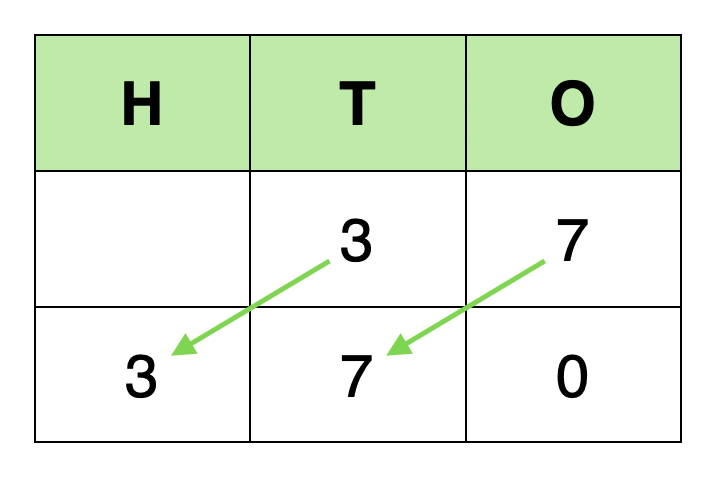# Multiplying by 10, 100 and 1000

## Multiplying by 10

Let's look at the following example:

37 x 10 = 370

When a number is multiplied by 10, it looks like all that happens is that a zero is added to the number that is being multiplied. However, the place value of each of the digits has changed:When a number is multiplied by ten, all the digits move one place to the left.

The ones column cannot be left empty, so a zero is placed there.

## Multiplying by 100

Let's look at the following example:

37 x 100 = 3700

When a number is multiplied by 100, it looks like all that happens is that two zeros are added to the number that is being multiplied. However, the place value of each of the digits has changed:

When a number is multiplied by a hundred, all the digits move two places to the left.

The tens and ones columns cannot be left empty, so a zero is placed in each.

## Multiplying by 1000

Let's look at the following example:

37 x 1000 = 37000

When a number is multiplied by 1000, it looks like all that happens is that three zeros are added to the number that is being multiplied. However, the place value of each of the digits has changed:

When a number is multiplied by a thousand, all the digits move three places to the left.

The hundreds, tens and ones columns cannot be left empty, so a zero is placed in each.

## Multiplying decimals by 10, 100 and 1000

When multiplying a decimal number by 10, 100 or 1000, it might look like the decimal point is moving.

However, the decimal point does not move. As in the above examples, it is the place value of each of the digits that changes:

Let's look at the following examples:

3.275 x 10 =

3.275 x 100 =

3.275 x 1000 =

When a decimal number is multiplied by ten, all the digits move one place to the left. The decimal point does not move.

There are no thousandths in this answer, so it can be written without the final zero:

3.275 x 10 = 32.75

When a decimal number is multiplied by a hundred, all the digits move two places to the left. The decimal point does not move.

There are no hundredths or thousandths in this answer, so it can be written without the final two zeros:

3.275 x 100 = 327.5

When a decimal number is multiplied by a thousand, all the digits move three places to the left. The decimal point does not move.

There are no tenths, hundredths or thousandths in this answer, so it can be written without the decimal point:

3.275 x 100 = 3275

## National Curriculum

Multiplying by 10, 100 and 1000 appears as an objective in the Year 5 programme of study.

• multiply and divide whole numbers and those involving decimals by 10, 100 and 1000

However, from Year 2, children are expected to know the multiplication facts for the 10 times table, and it is likely that the skill of multiplying larger numbers by 10, as well as multiplying by 100, will be taught and practised prior to Year 5.